• Matlab 连通分量处理， 二维和三维 (Matlab, Connected Component Process, 2D&3D)The code for your reference, which is used to remove the minor components:function Connect_Elimi = Connection_Judge_3D...

Matlab 连通分量处理， 二维和三维 (Matlab, Connected Component Process, 2D&3D)

The code for your reference, which is used to remove the minor components:

function Connect_Elimi = Connection_Judge_3D(Binary_Img, reject_T)

Connect_Elimi = Binary_Img;

% find components

CC = bwconncomp(Binary_Img);

% component number

C_number = CC.NumObjects;

% pixel number

numPixels = cellfun(@numel, CC.PixelIdxList);

totalPixels_N = sum(numPixels);

% remove minor components

for k = 1:C_number

cPixel_N = length( CC.PixelIdxList{k} );

ratio = cPixel_N / totalPixels_N;

if ratio < reject_T

Connect_Elimi(CC.PixelIdxList{k}) = 0;

end

end展开全文• Matlab中有bwlabel\bwboundaries两个连通分量标记函数 bwlabel（二维0-1） 作用：Label connected components in 2-D binary image 语法 L = bwlabel(BW) L = bwlabel(BW,conn) [L,n] = bwlabel(___) 说明 L...

bwlabel（二维0-1）

作用：Label connected components in 2-D binary image

语法
L = bwlabel(BW)
L = bwlabel(BW,conn)
[L,n] = bwlabel(___)

说明

L = bwlabel(BW)返回标签矩阵L，其中包含BW中找到的8个连接对象的标签。
L = bwlabel(BW,conn)返回一个标签矩阵，其中conn指定连接，4或者8。
[L,n] = bwlabel()也返回n，即BW中找到的连接对象的数量。

对于bwlabel来说，其作用大致如下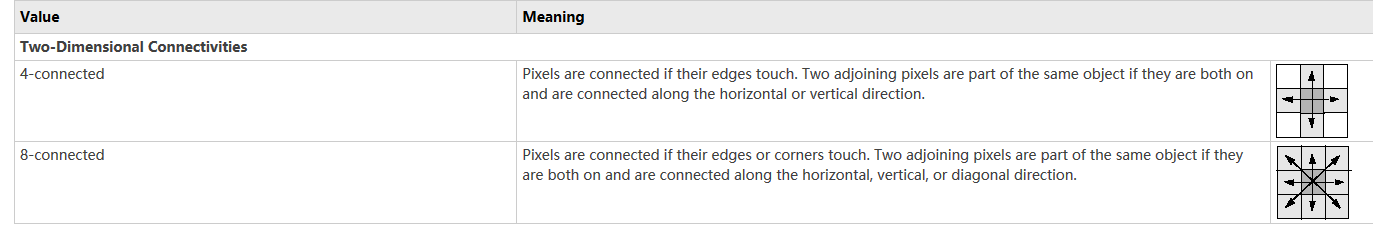bwlabeln（多维）

作用 Label connected components in binary image

语法
L = bwlabeln(BW)
L = bwlabeln(BW,conn)
[L,n] = bwlabeln(___)

说明

L = bwlabeln(BW) returns a label matrix, L, containing labels for the connected components in BW.

L = bwlabeln(BW,conn) returns a label matrix, where conn specifies the connectivity.

[L,n] = bwlabeln(___) also returns n, the number of connected objects found in BW.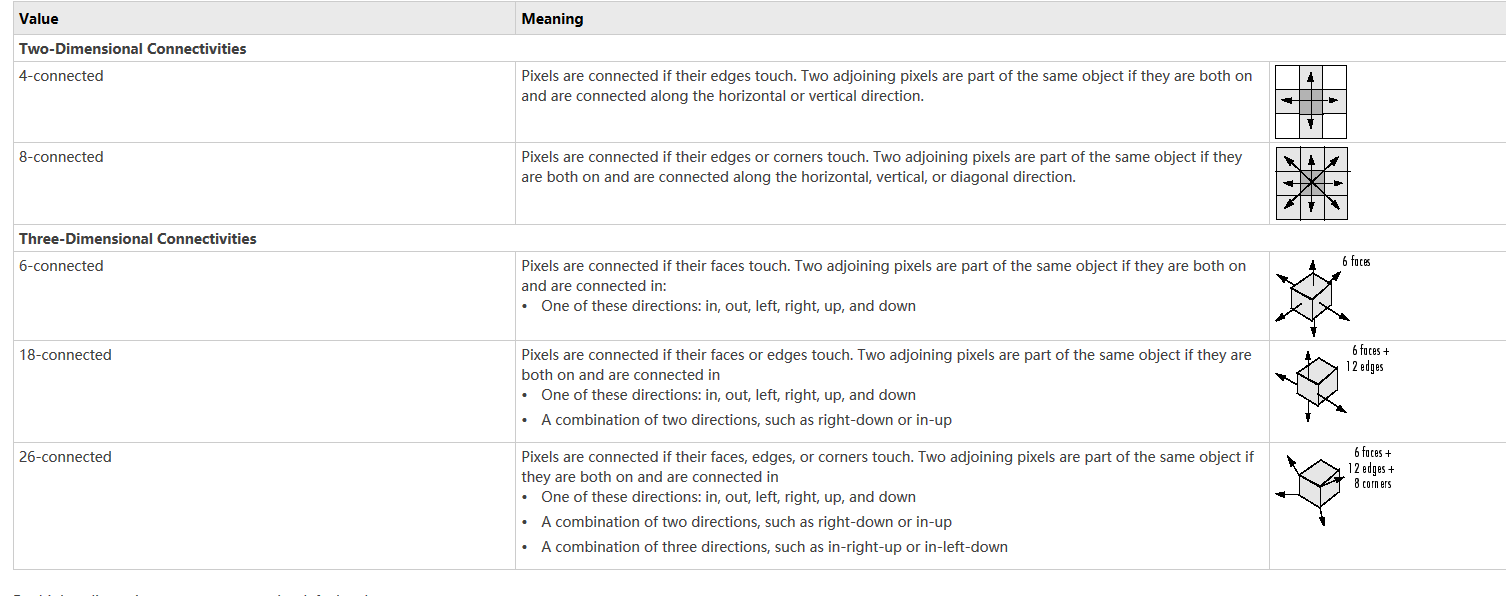bwboundaries

意译为：跟踪二进制图像中的区域边界

语法
B = bwboundaries(BW)
B = bwboundaries(BW,conn)
B = bwboundaries(BW,conn,options)
[B,L]= bwboundaries(___)
[B,L,n,A] = bwboundaries(___)

BW — Input binary image
conn — Pixel connectivity ,8 (default) | 4
options — Determine whether to search for both parent and child boundaries,‘holes’ (default) | ‘noholes’
B — Row and column coordinates of boundary pixels(边界像素的行坐标和列坐标)
L — Label matrix
n — Number of objects found
A — Parent-child dependencies between boundaries and holes(square, sparse, logical matrix.正方形，稀疏，逻辑矩阵)
说明
B = bwboundary (BW)在二值图像BW中，追踪物体的外部边界，以及物体内部的孔边界。bwboundary也向下延伸到最外层的对象(父对象)并跟踪其子对象(父对象完全包围的对象)。返回边界像素位置的单元格数组B。
B = bwboundary (BW,conn)跟踪对象的外部边界，其中conn指定在跟踪父和子边界时使用的连接。
B = bwboundary (BW,conn,options)跟踪对象的外部边界，其中的选项要么是“hole”，要么是“noholes”，指定是否希望包含其他对象内部的hole边界。
[B,L]= bwboundaries()返回一个标签矩阵L，其中标记了对象和孔。
[B,L,n,A] = bwboundaries()(_)返回找到的对象数量n和邻接矩阵A。

例子

//在图像上覆盖区域边界

//Convert grayscale image to binary image using local adaptive thresholding.
BW = imbinarize(I);

//Calculate boundaries of regions in image and overlay the boundaries on the image.
[B,L] = bwboundaries(BW,'noholes');

imshow(label2rgb(L, @jet, [.5 .5 .5]))
hold on
for k = 1:length(B)
boundary = B{k};
plot(boundary(:,2), boundary(:,1), 'w', 'LineWidth', 2)
end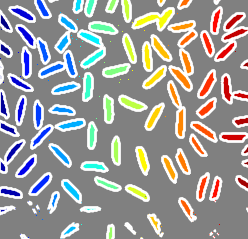//在图像上覆盖区域边界，并用区域号标注

//Calculate boundaries of regions in the image.

[B,L,N,A] = bwboundaries(BW);

//Display the image with the boundaries overlaid.
//Add the region number next to every boundary (based on the label matrix). Use the zoom tool to read individual labels.

imshow(BW); hold on;
colors=['b' 'g' 'r' 'c' 'm' 'y'];
for k=1:length(B),
boundary = B{k};
cidx = mod(k,length(colors))+1;
plot(boundary(:,2), boundary(:,1),...
colors(cidx),'LineWidth',2);

%randomize text position for better visibility
rndRow = ceil(length(boundary)/(mod(rand*k,7)+1));
col = boundary(rndRow,2); row = boundary(rndRow,1);
h = text(col+1, row-1, num2str(L(row,col)));
set(h,'Color',colors(cidx),'FontSize',14,'FontWeight','bold');
end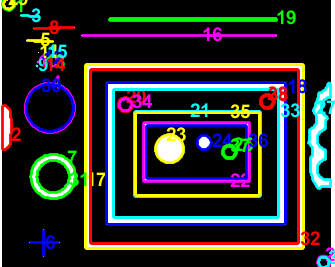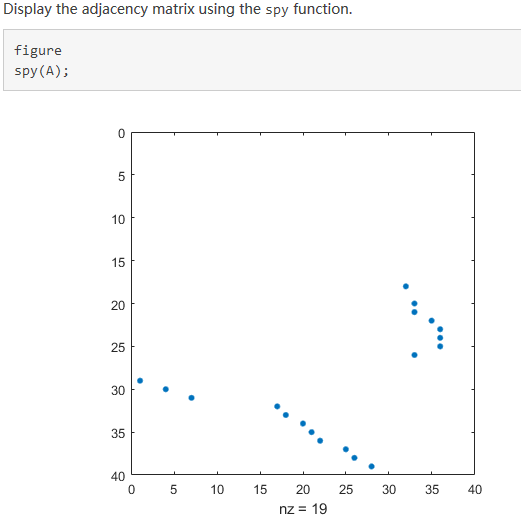//以红色显示对象边界，以绿色显示孔边界

//Calculate boundaries.

[B,L,N] = bwboundaries(BW);
//Display object boundaries in red and hole boundaries in green.

imshow(BW); hold on;
for k=1:length(B),
boundary = B{k};
if(k > N)
plot(boundary(:,2), boundary(:,1), 'g','LineWidth',2);
else
plot(boundary(:,2), boundary(:,1), 'r','LineWidth',2);
end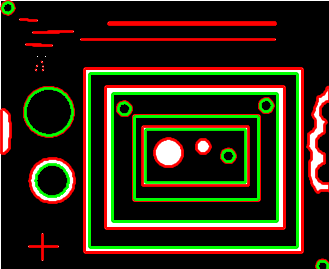好用的网站：https://ww2.mathworks.cn/help/images/ref/bwboundaries.html?s_tid=doc_ta
比买书有用多了，我买过一本matlab完全自学一本通，其实还不如这个网站来得直接。
openCV上也有这样得功能函数。

展开全文• matlab连通分量去除孤立点

千次阅读 2019-06-25 17:09:27
%显示最大连通区域， [~, max_id] = max(areas); max_rect = rects(max_id, :); % show the largest connected region % figure(2), % imshow(bw_img); % rectangle('position', max_rect, 'EdgeColor', 'r'); bw...
src_img_name = 'haixing.png';

% get binary image
gray_img = rgb2gray(img);
T = graythresh(gray_img);
bw_img = imbinarize(gray_img, T);

% find the largest connected region
img_reg = regionprops(bw_img,  'area', 'boundingbox');
areas = [img_reg.Area];
rects = cat(1,  img_reg.BoundingBox);

figure(1),
imshow(bw_img);
for i = 1:size(rects, 1)
rectangle('position', rects(i, :), 'EdgeColor', 'r');
end

%显示最大连通区域，
[~, max_id] = max(areas);
max_rect = rects(max_id, :);

% show the largest connected region
% figure(2),
% imshow(bw_img);
% rectangle('position', max_rect, 'EdgeColor', 'r');

bw_img2 = bwareaopen(bw_img,P);  %删除二值图像BW中面积小于P的对象，默认情况下conn使用8邻域
figure;
imshow(bw_img2);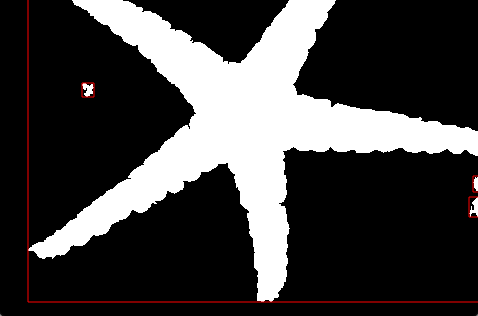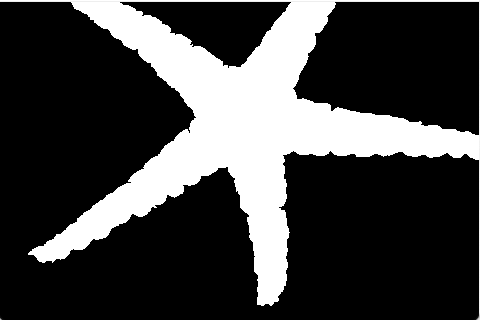展开全文matlab 图像分割
• 为了满足棒材计数在工业生产的实际应用需求,提出了一种基于提取连通分量的算法，以实现目标棒材计数区域的自动定位，并对定位的区域采用提取连通分量算法实现对轮廓的标注，最后提出了一种区域轮廓周长的校正方法,...

FindConnectedPoint函数如下：

%保存边界坐标对象

Obj=[];

%以p为中心点，从边界图中取出3*3大小的块区域

Obj=p;

%将图像中的p点置零

%将块区域中的p点置零

Block(n_l+1,n_l+1)=0;

A=cell(1,3);

%将p点从下标矩阵中删除

for j=1:size(ind,1)

if ind(j,:)==p

ind(j,:)=[];

break;

end

end

%寻找块区域中为1的点的下标

[rows,cols]=find(Block==1);

ind_sub=cat(2,rows,cols);

if ~isempty(ind_sub)

%确定块中数值为1的点的坐标

for i=1:size(ind_sub,1)

p_next=[];

if ind_sub(i,1)<=n_l+1

p_next(1,1)=p(1,1)-abs(n_l+1-ind_sub(i,1));

if ind_sub(i,2)<=n_l+1

p_next(1,2)=p(1,2)-abs(n_l+1-ind_sub(i,2));

else

p_next(1,2)=p(1,2)+abs(n_l+1-ind_sub(i,2));

end

else

p_next(1,1)=p(1,1)+abs(n_l+1-ind_sub(i,1));

if ind_sub(i,2)<=n_l+1

p_next(1,2)=p(1,2)-abs(n_l+1-ind_sub(i,2));

else

p_next(1,2)=p(1,2)+abs(n_l+1-ind_sub(i,2));

end

end

Obj=cat(1,A{1,1},Obj);

end

%更新下标矩阵

ind=A{1,2};

end

end

%返回结果

end

京ICP备09083238号

展开全文• Matlab中bwlabel函数的使用 bwlabel 但bwlabel函数有个问题是，他只区分0和1两种情况，举个例子： a = 1 1 1 2 2 2 0 0 0 bwlabel(a,4) = 1 1 1 1 1 1 0 0 0 所以他判定一二行都是1，所以是联通的，无matlab 图像识别
• 实现用于查找有向图的强连通分量的 Tarjan 算法。 在强连通分量 (SCC) 中，每个节点到每个其他节点都有一条路径。 SCC 是不相交的。 入度或出度为零或属于无环图的节点自己形成 SCC。 接受邻接矩阵作为输入。 为了...matlab
• 该函数返回 ND 逻辑数组（与输入数组大小相同），其中设置了 n 个最大的连通分量索引。 输入： ------- Xin - 输入 ND 阵列。 必需的。 conn - 连通性定义，可以是标量或连通性数组，维数与输入数组相同。 如果为...matlab
• 连通分量的提取

千次阅读 2017-02-28 22:34:53
应用背景：在许多自动图像分析应用中，如何识别出图像中的不同之处，往往是从其二值图像中提取连通分量连通分量的定义：令S是一个像素子集，如果S中的全部像素之间存在一个通路（m通路或8通路），则可以说两个...图像处理 matlab 连通分量
• matlab学习——强连通分量

千次阅读 2018-05-07 15:44:54
最近motion graph相关实验，发现实现运动过渡需要构建运动图，而为了避免运动过渡陷入死胡同，需要对图结构进行裁剪，方法就是计算图模型的极大强联通分量，但是自己懒得去实现，所以就去搜了一下matlab中有无相关...matlab
• 保留最大连通区域后，如何进行孔洞填充，孩子没学过多少matlab，简单解法就好matlab 有问必答
• LABEL 是 BWLABEL 的推广：BWLABEL 仅适用于二维二进制图像，而 LABEL 适用于任何类别的二维数组。...标记为 0 的像素是背景（对应于输入数组的 NaN 分量）。 标记为 1 的像素构成一个对象，标记为 2 的像素构matlab
• matlab连通区域的提取

热门讨论 2013-06-24 10:55:51
利此用代码进行图像的连通域的提取，简单通俗易懂，很好的选择
• 这个程序是一个图像处理的例子，以获得最大的连通分量。 有关更多信息，您可以通过我的电子邮件与我联系：shahab.jafari@gazikent.edu.trmatlab
• 形态学之连通分量提取

千次阅读 2018-10-06 20:34:45
• B=最大成分(A) 此功能查找网络中最大的连接组件。 输入 A 是网络的邻接矩阵。 输出 B 是最大组件中的节点列表。 A（B，B）将给予调整。 最大分量的矩阵。matlab
• 关于bwconncomp连通分量的BoundingBox问题。最近越来越懒了，能找到现成的就绝不手撸……而在做连通域分割的过程中，计算结果始终与预计的有出入。经过测试才弄明白BoundingBox输出的含义。总结分享一波matlab 算法 开发语言
• L = bwlabel(BW,n) 返回一个和BW大小相同的L矩阵，包含了标记了BW中每个连通区域的类别标签，这些标签的值为1、2、num(连通区域的个数)。n的值为4或8，表示是按4连通寻找区域，还是8连通寻找，默认为8。 四连通或八...
• ISLANDS 查找矩阵中所有 4 连通分量的“岛”。 该函数将返回三个输出参数，包括输入矩阵中最大的连接岛。 有关所有说明，请参阅详细帮助。 灵感来自最近发布的益智游戏： ...matlab
• 孔洞填充及连通分量提取

千次阅读 2018-05-24 21:03:49
（2） 实现连通分量的提取并计算连通分量的个数及每个连通分量的像素数。二、 代码思路（1）孔洞填充：先选取十字形结构作为结构元；接着生成一个由0组成的阵列X，大小与二值图像A相同，随机选取A中一个灰度值为1...孔洞填充 连通分量提取 MATLAB
• bwconncomp() 确定二值图像的连通分量，但图像实际上可能代表周期性域上的数据。 在这种情况下，CC2periodic() 通过沿图像边界扫描像素并合并出现在边界任一侧的对象来适当地转换 bwconncomp() 的输出。matlab
• ﻿﻿ 1 %% 第9章 形态学处理 2 3 %% imdilate膨胀 4 clc 5 clear 6 7 A1=imread('.\images\dipum_images_ch09\Fig0906(a)(broken-text).tif'); 8 info=imfinfo('.\images\dipum_images_ch09\Fig0906(a)(broken-...
• 根据邻接矩阵求最大连通分支的MATLAB函数，输入为邻接矩阵，输出为最大连通分支点集。
• 四、强连通分量连通分量：对于有向图G=(V,E)，强连通分量是一个最大节点集合C⊆V，对于该集合中的任一节点对u和v，路径u->v和路径v->u同时存在，即节点u和节点v可以互相到达。 强连通分量是深度优先搜索的一个...
• - PAGE PAGE 3 欢迎下载 该算法主要是仿照c中利用先深搜索算法求图的连通分量的算法改写的 该算法假设有20个点1号和24号相连2号和3号相连5号和67号相连8号和9号相连其他点都是孤立点 结果图如下 代码如下 clear N_......

matlab连通分量matlab 订阅